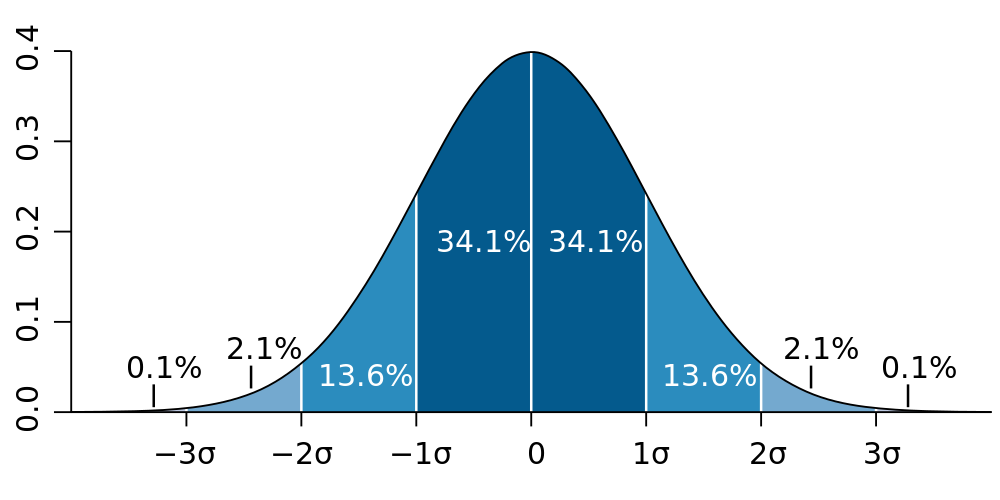# Mean and variance of a random variable

03/01/2021

## Instructions:

Use the left and right arrow keys to navigate the presentation forward and backward respectively. You can also use the arrows at the bottom right of the screen to navigate with a mouse.

FAIR USE ACT DISCLAIMER:
This site is for educational purposes only. This website may contain copyrighted material, the use of which has not been specifically authorized by the copyright holders. The material is made available on this website as a way to advance teaching, and copyright-protected materials are used to the extent necessary to make this class function in a distance learning environment. The Fair Use Copyright Disclaimer is under section 107 of the Copyright Act of 1976, allowance is made for “fair use” for purposes such as criticism, comment, news reporting, teaching, scholarship, education and research.

## Outline

• The following topics will be covered in this lecture:

• Mean, median and mode
• Weighted mean
• Mean of a frequency distribution
• Mean of a probability distribution
• Standard deviation
• Variance

## Motivation

• Our goal in this course is to use statistics from a small, representative sample to say something general about the larger, unobservable population or phenomena.

• Recall, the measures of the population are what we referred to as parameters.

• Parameters are generally unknown and unknowable.

• For example, the mean age of every adult living in the United States is a parameter for the adult population of the USA.
• We cannot possibly know this value exactly as there are people who cannot be surveyed and / or don't have accurate records.
• If we have a representative sample we can compute the sample mean.
• The sample mean will almost surely not equal population mean, due to the natural variation (sampling error) that occurs in any given sample.
• However, if we have a good probabilistic model for the ages of adults, we can use the sample statistic to estimate the general, unknown population parameter.
• Random variables and probability distributions give us the model for estimating population parameters.

• Note: we can only “find” the parameters exactly in very simple examples like games of chance.

• Generally, we will have to be satisfied with estimates of the parameters that are uncertain, but also include measures of “how uncertain”.

## Characteristics of dataCourtesy of M. W. Toews CC via Wikimedia Commons.

• In statistics, we try to characterize data and populations by a number of the features that they exhibits.
• For a single variable, the most common measures are:
1. Center: A representative value that indicates where the middle of the data set is located.
2. Spread: A measure of the amount that the data values vary around the center.
• We will now recall some measures of center:
1. mean;
2. median; and
3. mode.
• Each of these usually gives a different view of where the “most central point” of the data lies.

## Mean

• The (arithmetic sample) mean is usually the most important measure of center.

• Suppose we have a sample of $$n$$ total measurements of some random variable $$X$$.

• We will denote these measurements $$x_1, x_2, \cdots, x_n$$
• Then, the (arithmetic sample) mean is defined

$\text{Sample mean} = \overline{x} = \frac{x_1 +x_2 +\cdots + x_n}{n}= \frac{\sum_{i=1}^n x_i}{n}$

• Q: is the sample mean a statistic or a parameter?

• A: the sample mean is computed from a sample and thus is a statistic.
• For this reason, if we took new measurements from a new sample of the population, we could get a different value.
• The random difference between the sample mean and the mean of the true population mean is called sampling error.
• An important property of the sample mean is that it tends to vary less over re-sampling than other statistics.

• That is, it tends to stay close to the same value.
• However, the sample mean is very sensitive to outliers.

• If outliers exist in the data, the mean can be drawn far away from the “main” cluster of data.
• A statistic is called resistant if it doesn't change very much with respect to outlier data.

## Median and mode

• A different notion of center is the middle of the data.
• For a numerical measurement, we can always order the data so that we go from low to high or high to low.
• Median – the median is the middle of the ordered data set.
• If there are an odd number of measurements, the median is defined as the middle value exactly.
• If there are an even number of measurements, we split the data into the lower $$50\%$$ and upper $$50\%$$ of the measurements;
• then we take the median to be the mean of the:
1. largest of the lower $$50\%$$; and
2. smallest of the upper $$50\%$$.
• Another notion of the most “central point” in the data can be the value that is measured most frequently.
• Mode – the mode is the observed value that is most frequent in the data.